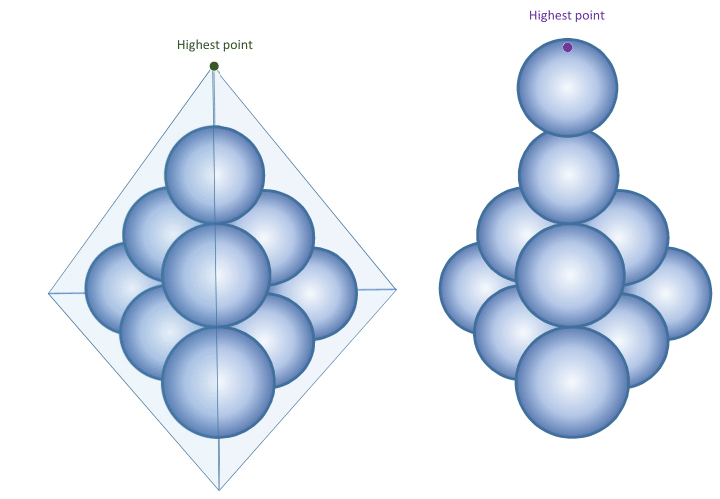# 2017-03-06 Advanced

What is the largest positive integer $n$, that is not a multiple of 10, such that

$\Large \left \lfloor \frac{n^2}{100}\right \rfloor$

is a perfect square?

Notation: $\lfloor \cdot \rfloor$ denotes the floor function.

In the $xyz$-coordinate system, a massive particle is initially at rest on a plane whose equation is given below: $x + 2y + 3z = 0.$ The particle is launched with initial velocity $(v_x,v_y,v_z) = (-3,7,12)$. It travels under the influence of a gravitational acceleration of $(a_x,a_y,a_z) = (0,0,-10)$ until it intersects the plane once more.

When the particle intersects the plane for the second time, how far away is it from the launch point (to 3 decimal places)?Ten identical spheres are packed as above. You can either encase the entire stack in a tightly fitting tetrahedron, or you can balance one additional sphere on top. Which will be higher off the ground, the top vertex of the tetrahedron or the top of the additional sphere?Details

• Each of the spheres in the top two layers of the original arrangement is supported by three spheres which are all tangent to one another.

• The entire arrangement sits on a level ground.

• Each face of the encasing tetrahedron is tangent to six of the spheres in the stack.

• The additional sphere is identical to the others, is supported only by a single sphere below it to which it is tangent, and its center is directly above the center of the sphere below it.

Top image credit: Etsy.comConsider all rhombuses with side length 2 whose centers are the origin and vertices lie on the coordinate axes. The area that envelopes these rhombuses can be expressed as $\pi r$. What is $r$ to 2 decimal places?When we place $1 \times 2$ dominoes on a board that isn't completely covered, sometimes we are able to move a domino horizontally or vertically. For example, in this $3 \times 2$ board, the yellow domino can move horizontally while the blue domino is stuck.

Consider an $8 \times 8$ board tiled with several $1 \times 2$ dominos. What is the minimum number of dominos for which there exists an arrangement in which no domino can move (horizontally or vertically) into another position?

Details and Assumptions:

• The dominos are not allowed to stick out of the board or overlap.
• We get to choose the placement of the dominos.
×

Problem Loading...

Note Loading...

Set Loading...